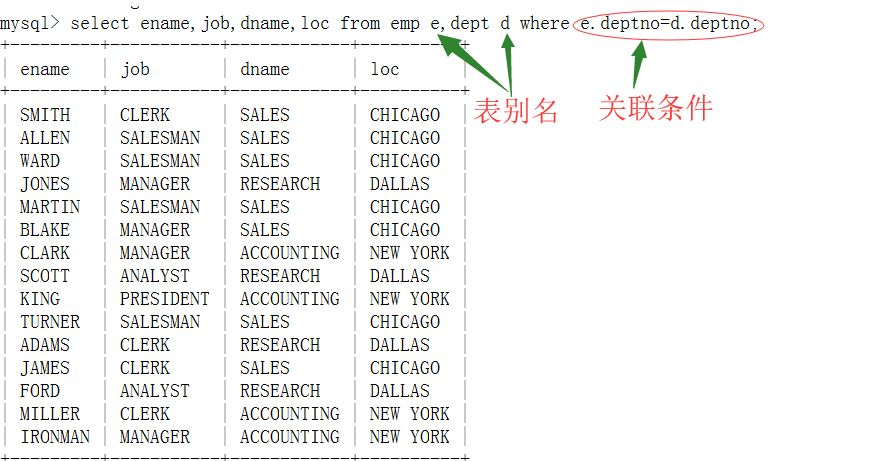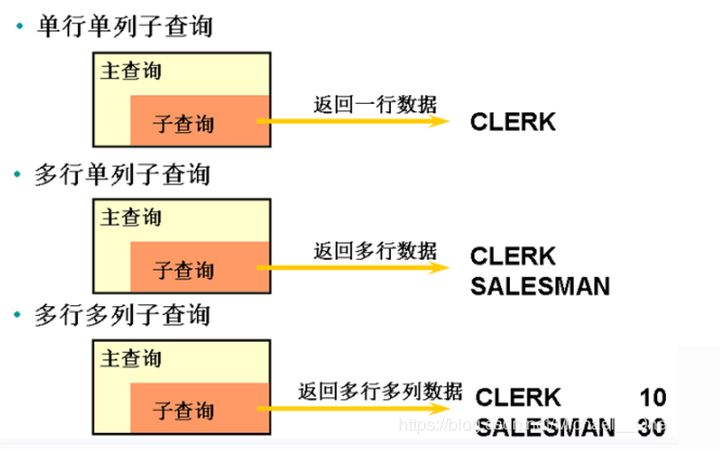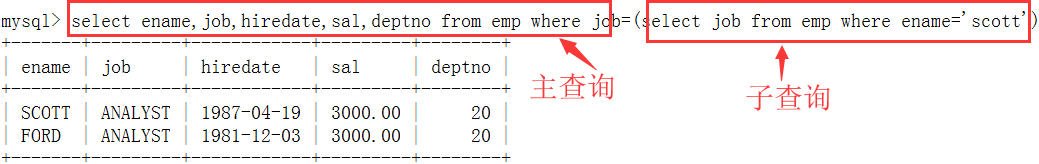# MySQL关联查询

1、概念

2、笛卡尔积3、等值连接4、内连接5、外连接

mysql外连接只支持左外连接，右外连接，不支持全外连接

左外连接:

PS:mysql 不支持全外连接

select e.ename,e.job,e.deptno,d.dname from emp e left join dept d on e.deptno = d.deptno;

6、自连接

mysql > select a.ename 员工姓名,
a.job 员工职位,
b.ename 上司姓名,
b.job 上司职位
from emp a join emp b on a.mgr=b.empno;1)子查询可以在where子句中
2)子查询可以在from子句中
3)子查询可以在having子句中
4)子查询可以在select字句中，相当于外连接的另外一种写法。
1、在where子句中

select ename,job,hiredate,sal,deptno from emp where job=(select job from emp where ename='scott');mysql > select ename,job,hiredate,sal from emp where sal > (select avg(ifnull(sal,0)) from emp);

select ename,job,hiredate,sal,deptno from emp where deptno in (select distinct deptno from emp where job='salesman') and job <> 'salesman';

exists 关键字

mysql > select deptno,dname,loc from dept d where exists (select * from emp e where d.deptno =e.deptno);

2、在from子句中
from子句用于指定表，如果想在一个子查询的结果里继续查询，则子查询需要写在from子句中，相当于一个表。

mysql > select e.ename,e.sal,t.avg_sal,t.deptno from emp e join (select deptno,avg(ifnull(sal,0)) 'avg_sal' from emp group by deptno) t on e.deptno = t.deptno and e.sal>t.avg_sal order by t.deptno;

select e.ename, e.sal, t.avg_sal from emp e , (select deptno,avg(ifnull(sal,0)) 'avg_sal' from emp group by deptno) t where e.deptno = t.deptno order by t.deptno;
3、在having子句中

mysql > select deptno,avg(ifnull(sal,0)) from emp group by deptno having avg(ifnull(sal,0))>(select avg(ifnull(sal,0)) from emp where deptno=30);
4、在select子句中

select ename,sal,
(select avg(ifnull(sal,0)) from emp a where a.deptno=b.deptno) avg_sal ,
(select sum(sal) from emp c where c.deptno=b.deptno ) sum_sal
from emp b order by b.deptno;

EXISTS

SELECT 字段 FROM table WHERE EXISTS (subquery);

subquery是一个受限的SELECT语句（不允许有COMPUTE子句和INTO关键字）

SELECT * FROM A WHERE EXISTS (SELECT 1 FROM B WHERE B.id = A.id);

EXISTS执行顺序：

1、首先执行一次外部查询，并缓存结果集，如 SELECT * FROM A

2、遍历外部查询结果集的每一行记录R，代入子查询中作为条件进行查询，如 SELECT 1 FROM B WHERE B.id = A.id

3、如果子查询有返回结果，则EXISTS子句返回TRUE，这一行R可作为外部查询的结果行，否则不能作为结果

NOT EXISTS

SELECT 字段 FROM table WHERE NOT EXISTS (subquery);

subquery是一个受限的SELECT语句（不允许有COMPUTE子句和INTO关键字）

SELECT * FROM A WHERE NOT EXISTS (SELECT 1 FROM B WHERE B.id = A.id);
NOT EXISTS执行顺序：

1、首先执行一次外部查询，并缓存结果集，如 SELECT * FROM A

2、遍历外部查询结果集的每一行记录R，代入子查询中作为条件进行查询，如 SELECT 1 FROM B WHERE B.id = A.id

3、如果子查询没有返回结果（与EXISTS相反），则NOT EXISTS子句返回TRUE，这一行R可作为外部查询的结果行，否则不能作为结果

IN
IN常用于where表达式中，其作用是查询某个范围内的数据。

select * from where field in (value1,value2,value3,…)
NOT IN

select * from where field not in (value1,value2,value3,…)

student：学生表，其中有字段sno为学号，sname为学生姓名

course：课程表，其中有字段cno为课程号，cname为课程名称

student_course_relation：选课表，记录学生选择了哪些课程，其中有字段sno为学号，cno为课程号

1、在子查询中使用NULL，仍然返回结果集

select * from student;
select * from student where exists (select 1);
select * from student where exists (select null);

2、EXISTS子查询返回的是一个布尔值true或false

EXISTS用于检查子查询是否至少会返回一行数据，该子查询实际上并不返回任何数据，而是返回布尔值true或false，EXISTS指定一个子查询，检测行的存在。

EXISTS只在乎子查询中是否有记录，与具体的结果集无关，所以下面示例中，子查询中的select sno也可以换成select cno或者select 1，查询出的结果集是一样的。

select * from student a
where exists (select sno from student_course_relation b where b.cno=3 and b.sno=a.sno);

select * from student a
where exists (select cno from student_course_relation b where b.cno=3 and b.sno=a.sno);

select * from student a
where exists (select 1 from student_course_relation b where b.cno=3 and b.sno=a.sno);

3、比较使用EXISTS和IN的查询

select * from student
where exists (select sno from student_course_relation where cno=1 and student_course_relation.sno=student.sno)

select * from student
where sno in (select sno from student_course_relation where cno=1)

EXISTS查询：先执行一次外部查询，然后为外部查询返回的每一行执行一次子查询，如果外部查询返回100行记录，sql就将执行101次查询。

IN查询：先查询子查询，然后把子查询的结果放到外部查询中进行查询。IN语句在mysql中没有参数个数的限制，但是mysql中sql语句有长度大小限制，整段最大为4M。IN引导的子查询只能返回一个字段。

4、比较使用NOT EXISTS和NOT IN的区别

select * from student a
where not exists (select sno from student_course_relation b where cno=1 and a.sno=b.sno)

select * from student a
where sno not in (select sno from student_course_relation b where cno=1)

NOT EXISTS：先执行一次外部查询，然后为外部查询返回的每一行记录R执行一次子查询，如果子查询没有返回记录，则NOT EXISTS子句返回TRUE，这一行R可作为外部查询的结果行。

NOT IN：外部查询在表中查询每条记录，符合要求的就返回结果集，不符合的就继续查询下一条记录，直到把表中的记录查询完，也就是说为了证明找不到，需要查询全部记录才能证明，NOT IN不会用到索引。

5、在插入记录前，检查这条记录是否存在，只有当记录不存在时才执行插入操作

insert into student_course_relation(sno, cno)
select '3' as sno, '2' as cno from student_course_relation
where not exists (select sno from student_course_relation where sno=3 and cno=2) limit 1

6、查询出选修了全部课程的学生姓名

select sname from student where sno in (
select sno from student_course_relation
group by sno -- 根据sno分组，统计每个学生选修了几门课，如果等于course的总数，就是我们要找的sno
having(count(sno)) = (select count(*) from course)
)

select cname from course
where not exists -- 找不到的记录，提交course
(select cno from student_course_relation where student_course_relation.cno=course.cno and sno='3')

select sname from student
where not exists ( -- 查询 没有 没有选修的课程 的学生，即选修了全部课程的学生
select cname from course -- 查询某学生没有选修的课程
where not exists
(select cno from student_course_relation
where student_course_relation.cno=course.cno and student_course_relation.sno=student.sno)
)

7、查询没有选择所有课程的学生

select * from student a
where exists (
select * from course b
where not exists (select * from student_course_relation c where c.sno=a.sno and c.cno=b.cno))

8、查询至少选修了学生3选修的全部课程的学生名单

select * from student m where sno!=3 and not exists (
select sno from student_course_relation x where sno=3 and not exists (
select cno from student_course_relation y where y.cno=x.cno and y.sno=m.sno
)
)

9、查询一门课也没有选的学生

select * from student a
where not exists (
select * from course b
where exists (select cno from student_course_relation c where c.cno=b.cno and c.sno=a.sno))

10、查询至少选修了一门课程的学生

select * from student a
where exists (
select cno from course b
where exists (select sno from student_course_relation c where c.cno=b.cno and c.sno=a.sno))

11、选出每门课程中成绩最高的学生

select * from student_course_relation a
where not exists (select * from student_course_relation b where b.cno=a.cno and b.score > a.score)

select * from student_course_relation a
where score=(select max(score) from student_course_relation b where b.cno=a.cno)

posted @ 2022-11-19 00:38  Endv  阅读(1521)  评论(0编辑  收藏  举报